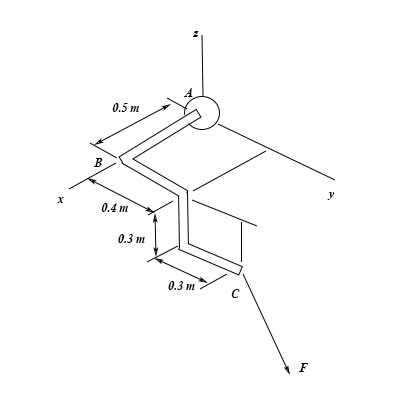# The pipe assembly is subjected to the force of F=\{550i+700j+450k\}N. Part A Determine the x,y z...

## Question:

The pipe assembly is subjected to the force of {eq}F=\{550i+700j+450k\}N. {/eq}

Part A

Determine the {eq}x,y, z {/eq} components of the moment of this force about point {eq}B. {/eq}

Express your answer to three significant figures separated by commas.## Vectors:

The physical quantities which require a definite magnitude along with a specific direction for a clear definition are known as the vector quantities. Force and moment are two examples of vector quantities.

## Answer and Explanation:

Given Data

• The force subjected at point C in Cartesian form is: {eq}\vec F = \left( {550\hat i + 700\hat j + 450\hat k} \right)\;{\rm{N}} {/eq}.

.

The coordinates of point C is,

{eq}C\left( {x,y,z} \right) = \left( {0.5,0.7, - 0.3} \right) {/eq}

The position vector of C with respect to origin is,

{eq}{\vec r_C} = 0.5\hat i + 0.7\hat j - 0.3\hat k {/eq}

The coordinates of point B is,

{eq}B\left( {x,y,z} \right) = \left( {0.5,0,0} \right) {/eq}

The position vector of B is,

{eq}{\vec r_B} = 0.5\hat i + 0\hat j + 0\hat k {/eq}

The relative position vector of BC is,

{eq}{\vec r_{BC}} = {\vec r_C} - {\vec r_B} {/eq}

Substitute the values in the above equation..

{eq}\begin{align*} {{\vec r}_{BC}} &= \left( {0.5\hat i + 0.7\hat j - 0.3\hat k} \right) - \left( {0.5\hat i + 0\hat j + 0\hat k} \right)\\ &= \left( {0.7\hat j - 0.3\hat k} \right)\;{\rm{m}} \end{align*} {/eq}

The moment due to the force is,

{eq}\vec M = {\vec r_{BC}} \times \vec F {/eq}

Substitute the values in the above equation.

{eq}\vec M = \left( {0.7\hat j - 0.3\hat k} \right) \times \left( {550\hat i + 700\hat j + 450\hat k} \right) {/eq}

{eq}\hat M=\begin{bmatrix} \hat i & \hat j & \hat k\\ 0&0.7 &-0.3\\ 550&700 &450 \end{bmatrix} {/eq}

Solve the above matrix,

{eq}\begin{align*} M &= \hat i\left( { 315 + 210} \right) - \hat j\left( {0 + 165} \right) + \hat k\left( {0 - 385} \right)\\ M &= \left( { 525\hat i - 165\hat j - 385\hat k} \right)\;{\rm{N}} \cdot {\rm{m}} \end{align*} {/eq}

Thus, the x-component of moment of force is {eq}525\;{\rm{N}} \cdot {\rm{m}} {/eq}, the y-component of moment of force is {eq}- 165\;{\rm{N}} \cdot {\rm{m}} {/eq} and the z-component of moment of force is {eq}- 385\;{\rm{N}} \cdot {\rm{m}} {/eq}.